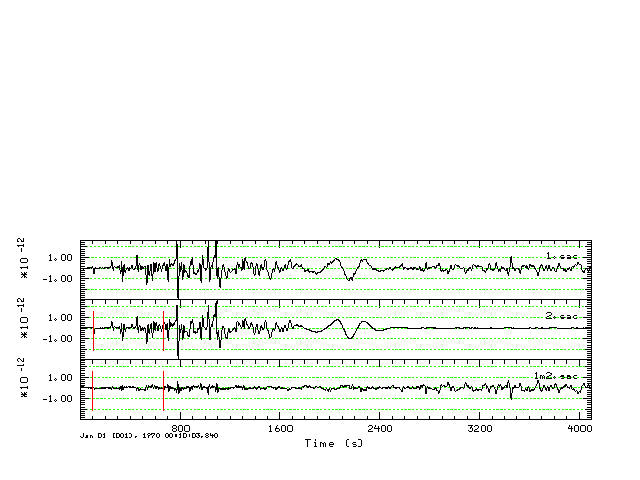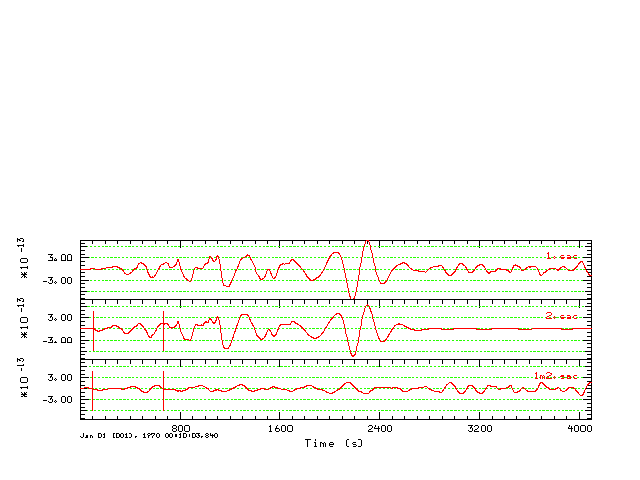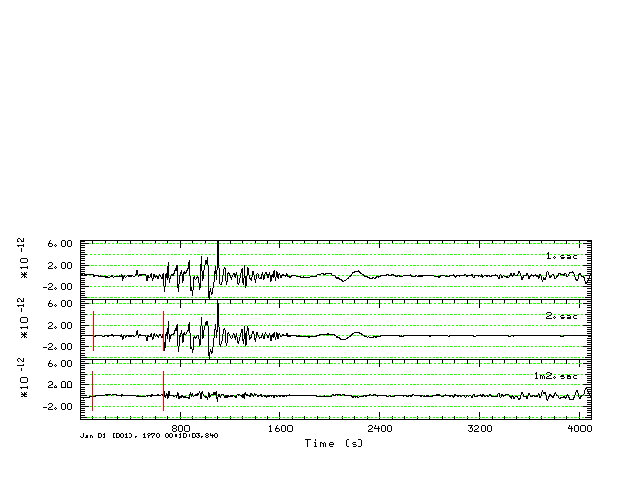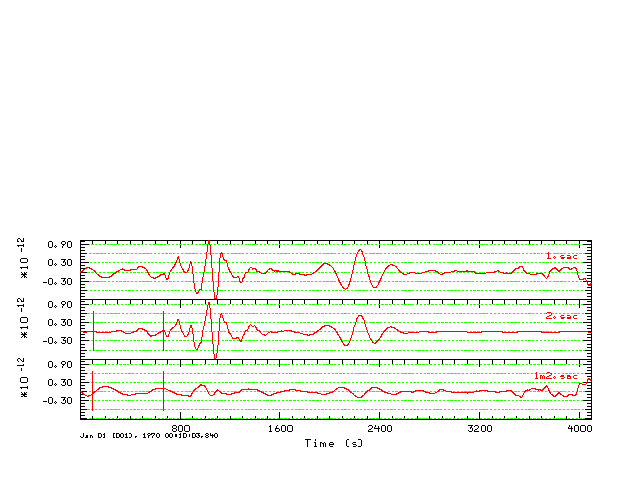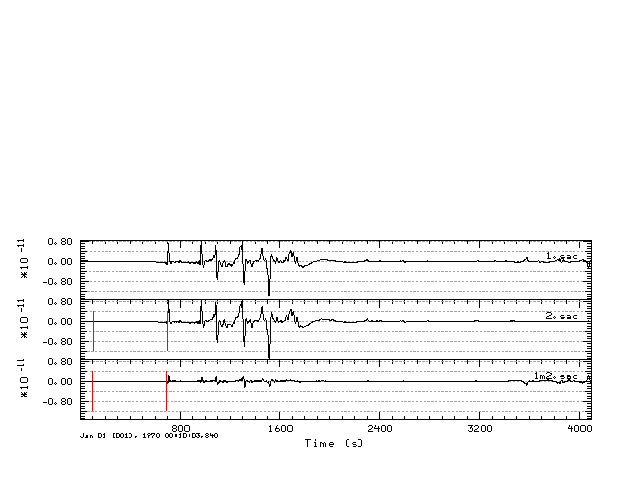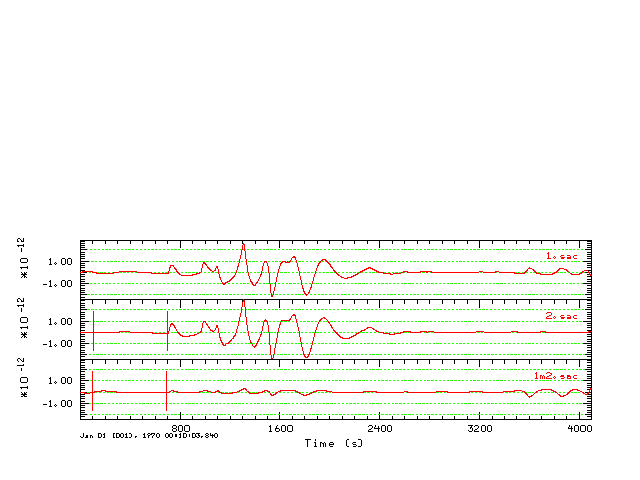## Introduction

This is a comparison between mineos and earth-flattened wavenumber integration synthetics (Computer Programs in Seismology - 3.30 ) for the AK-135f continental model.

I use the same model for both sets of synthetics, except that the wavenumber integration model does not have an inner core [The reason for this is that my formulation for wavenumber integration only permits fluid layers at the top or at the bottom of the elastic stack.

## Computations

The comparison is run in the directory MINEOS/share/mineos2.  The effort is computationally intensive in terms of computations and the construction of graphics. The objective is to compare the two sets of synthetics at arc distances of 30, 60 and 90 degrees for source depths of 10, 100 and 700 km.

The following steps are performed:

### 1. Wavenumber integration synthetics:

cd AK135-Z
zDOIT

After 10 hours of computation, the result will be a directory listing:

0100/     7000/  date.start  tak135sph.mod

The directory 0100 has the Green's functions for the source depth of 10.0 km, 1000 for a source depth of 100.0 km, and 7000 for a source depth of 700.0 km.
Within each directory are 10 Green's functions for each distance. For example, in the 1000 directory you will find

0333581000.RDD  0333581000.ZDS  0667171000.TDS  1000751000.RDS  1000751000.ZEX
0333581000.RDS  0333581000.ZEX  0667171000.TSS  1000751000.REX  1000751000.ZSS
0333581000.TDS  0667171000.RDS  0667171000.ZEX  1000751000.TSS
0333581000.TSS  0667171000.REX  0667171000.ZSS  1000751000.ZDD

The naming convention is distance_depth.green.

These synthetics have a sample rate of 2.0 sec, have a zero-phase  triangular source pulse of length 8 seconds.  This source pulse has a spectral zero at the Nyquist frequency and a corresponding spectral shaping for frequencies greater than 1/2 the Nyquist frequency.  The synthetics are those of ground velocicy in units of cm/sec.

### 2. Normal mode synthetics:

cd nDEMO6
DOIT

This runs the script DOEGN which computes the free oscillation frequencies and eigenfunctions for the spehroidal and toroidal modes.  The script is set to compute up to frequencies of 0.2 Hz (e.g., 200 mHz).  This computation will require several days.
After running DOEGN, SAC files are generated for the following cases:

`######       now compute the synthetics for a specific moment tensor that represents#       the following cases##       Source  STK     DIP     RAKE Mw [M0(dyne-cm)] Src-Rec Az#       DS      45      90      90   2.6 [1.0E+20]       0#       SS      22.5    90       0   2.6 [1.0E+20]       0#       DD      45      45      90   2.6 [1.0E+20]       0#       EX      Center of expansion  2.6 [1.0E+20]       0#####`
The SAC files are in the directories with names GRN_Syndat_DEP, where GRN is one of DS, SS, DD or EX and DEP is on of 010 (10 km), 100 (100 km) or 700 (700km).  The contents of these directories with files with names such as "Syndat.1969365: 0: 0:-4.170.LHZ.SAC". A zero phase source time function of a triangle with length 8 seconds is applied.  The SAC files are gound acceleration with units of nm/s/s.

When the evaluation is complete, run the script DOCLEAN to return the directory to the original distribution.

### 3. Populate the wavenumber integration synthetics

At this point, both sets of synthetics have the same sample interval and the same source time function.

In the directory MINEOS/share/mineos2  we will create subdirectories with names TEST.DEP.DEG where DEP is 010, 100 or 700 and DEG is 30, 50 or 90. The reason for this organization is that I wish to compare the two types of synthetics at the same depth and distance.

DOPOPULATERBH

This creates the dirrectories, within which there is a new directory called NEW_-Z with the CPS Green's functions. For example the command

rbh> ls -R TEST.010.030
TEST.010.030:
NEW_-Z/

TEST.010.030/NEW_-Z:
0333580100.RDD  0333580100.REX  0333580100.TDS  0333580100.ZDD  0333580100.ZEX

### 4. Apply the source mechanism to the wavenumber integration synthetics

DOPOPULATEMCH

This systematically creates the synthetics for the specific mechanism.
It  uses the program wvfmch96 to compute the predicted synthetics on the basis of the observed. In this case the observed are just the Z, R and T components of the SS Green's functions, relying on the fact that the SAC header variable AZ is set to 0.0 for the Green's functions.  The program wvfmch96 converts the Green's functions units to m/sec for the given moment of 1.0E+20 dyne-cm.

The result is

rbh> ls -R TEST.010.030TEST.010.030:
DD.WK/    DS.WK/  NEW_-Z/    SS.WK/

TEST.010.030/DD.WK:
D.-Z.LHR  D.-Z.LHT  D.-Z.LHZ

TEST.010.030/DS.WK:
D.-Z.LHR  D.-Z.LHT  D.-Z.LHZ

TEST.010.030/NEW_-Z:
0333580100.RDD  0333580100.REX  0333580100.TDS  0333580100.ZDD  0333580100.ZEX

TEST.010.030/SS.WK:
D.-Z.LHR  D.-Z.LHT  D.-Z.LHZ

Here the SS.WK are the wavenumber integration synthetics in units of m/s

### 5. Populate the free oscillation  synthetics

DOPOPULATEMIN

This script reads the selected synthetics, removes a linear trend, integrates and divides by 1.0E+09 to convert the synthetics in ground velocity in units of m/s. The result is

rbh> ls -R TEST.010.030

### 6. Compare the synthetics

DOALLHTML

This script searches through all distances and depths to run DOCMP which creates the .gif images for the documentation.
There are several aspects here.  First the wavenumber integration synthetics had a zero-phase source pulse with a duration of 4 seconds, while the free-oscillation synthetics had a zero phase source pulse with duration of 8.0 sec.

## Comparisons

### Timing

The first test compares the synthetized ground velocities for a source depth of 10 km and a distance of 90 degrees.  The focus here is on the amplitudes and the timing, especially that of the surface wave arrival.  For the distance the waveforms are bandpass filtered using the command

`    transfer from none to none freqlimits 0.001 0.002 0.06 0.10`
The figures compare the complete seismograms, e.g., a window of 700 to 3400 seconds after origin time, a focus on the surface-wave train using a window of 2200 to 3400 seconds after the origin time, and a zomm on the short period surface wave using a window 2800 to 3000 seconds after the origin time. The mineos synthetics are in red and the CPS synthetics are in blue.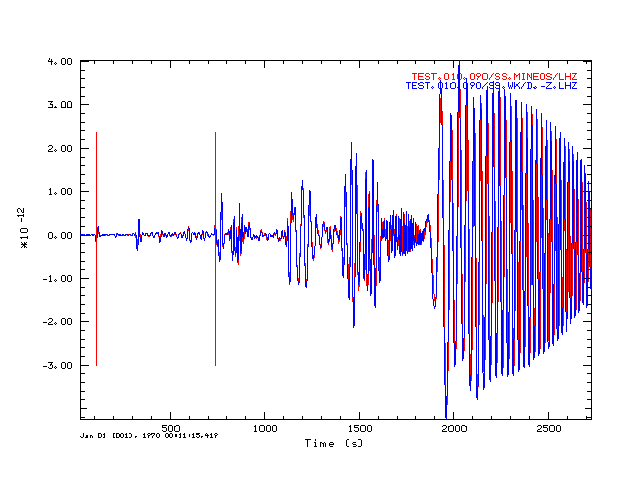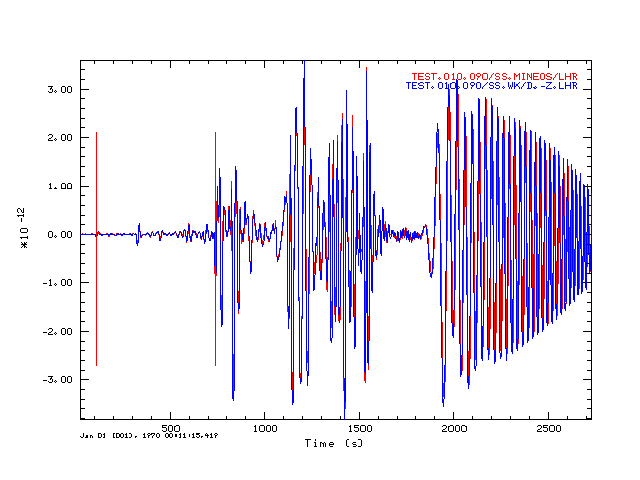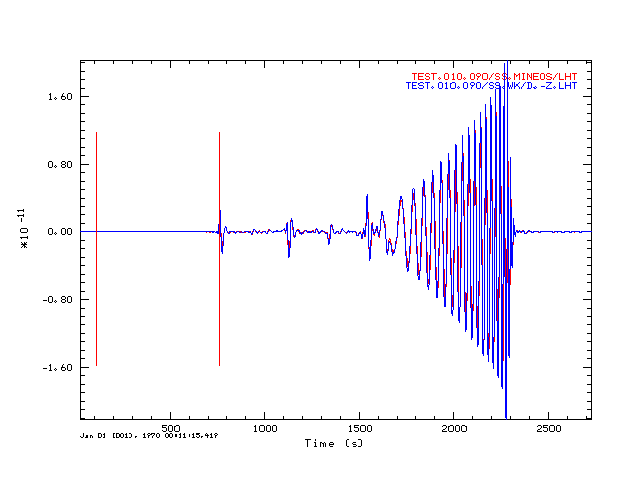Comparison of  mineos (red) and Earth flattened(blue) synthetics for the Z component at 90 degrees. The vertical red lines indicate the model predicted P- and S-times. Comparison of  mineos (red) and Earth flattened(blue) synthetics for the R component at 90 degrees. The vertical red lines indicate the model predicted P- and S-times. Comparison of  mineos (red) and Earth flattened(blue) synthetics for the T component at 90 degrees. The vertical red lines indicate the model predicted P- and S-times.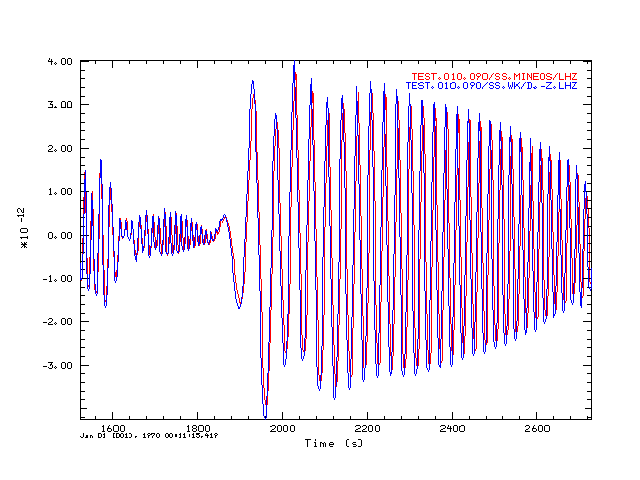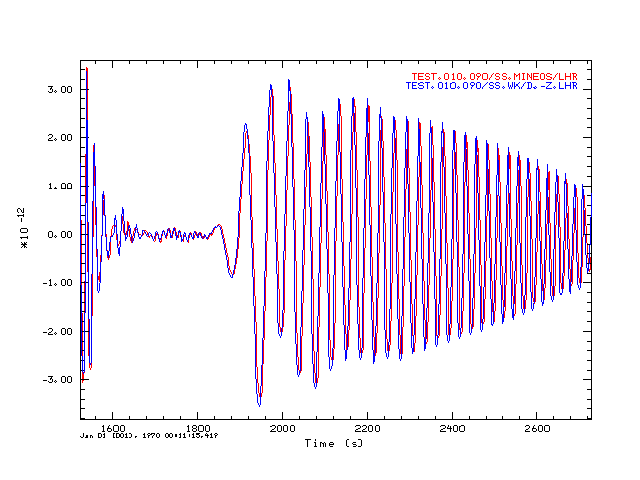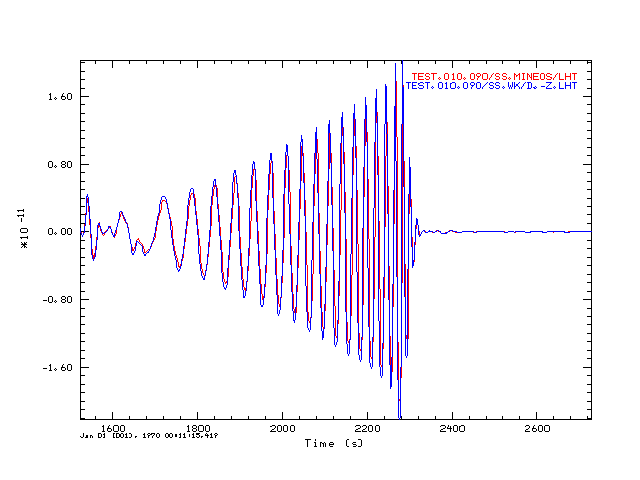Comparison of  mineos (red) and Earth flattened(blue) synthetics for the Z component at 90 degrees. This plot shows the arrivals in the travel time window of 2200 to 3400 seconds. Comparison of  mineos (red) and Earth flattened(blue) synthetics for the R component at 90 degrees. This plot shows the arrivals in the travel time window of 2200 to 3400 seconds.. Comparison of  mineos (red) and Earth flattened(blue) synthetics for the T component at 90 degrees. This plot shows the arrivals in the travel time window of 2200 to 3400 seconds..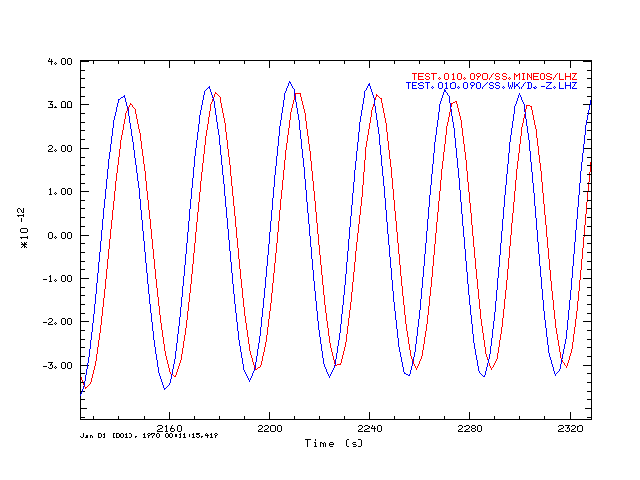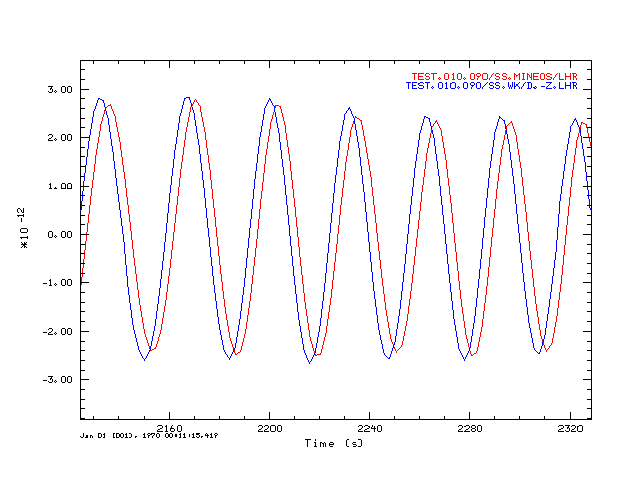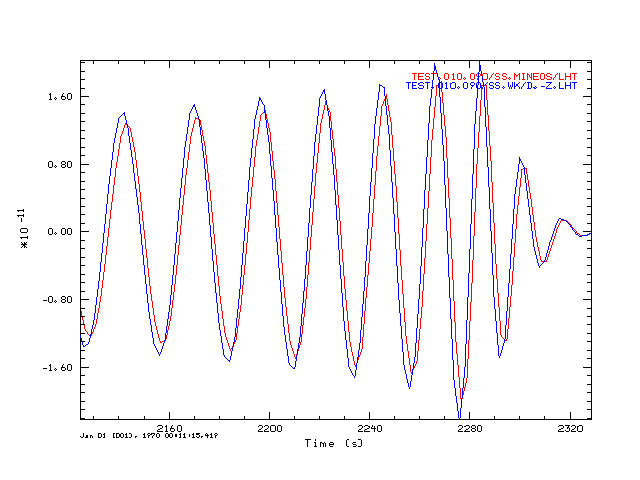Comparison of  mineos (red) and Earth flattened(blue) synthetics for the Z component at 90 degrees. This plot shows the arrivals in the travel time window of 2800 to 3000 seconds. This indicates a 3 second travel time difference at a 20 second period. Comparison of  mineos (red) and Earth flattened(blue) synthetics for the R component at 90 degrees. This plot shows the arrivals in the travel time window of 2800 to 3000 seconds. this indicates a 3 second travel time difference. Comparison of  mineos (red) and Earth flattened(blue) synthetics for the T component at 90 degrees. This plot shows the arrivals in the travel time window of 2800 to 3000 seconds. This indicates a 1 second travel time difference at a 20 second period.

### Strike-Slip synthetics as a function of depth

I created synthetics for strike-slip (SS), vertical dip-slip (DS) and 45 degree dip-slip (DD) mechanisms and compare the Z R and T components. Ground displacements are compared, which are filtered using the gsac command. This also serves as an independent test of the moment tensor formulation using in Computer Programs in Seismology.

`	transfer from none to none freqlimits 0.002 0.003 0.025 0.030`
which accounts for the fact that the minos synthetics only include frequencies as high as 0.10 HZ, whereas the wavenumber integration synthetics go up to 0.25 Hz. The use of the gsac transfer function is a simple way to accomplish a zero phase filter. This also eliminates much of the 0.03  rippling in the free-oscillation synthetics. isotropic source for completeness)

To permit the comparison I had to account for the fact that my wavenumber integrations synthetics had a zero-phase source pulse, whereas the mineos used a causal triangular pulse implemented in the frequency domain,

I also had to account for the fact that mineos accepts geodetic coordinates and then converts internally to geocentric. I had to slightly change my input to mineos so that the epicentral distance in kilometers agreed with that used for the wavenumber integration.

For each mechanism, source depth and arc distance, there are separate figures for the Z, R and T components. The traces shown are in two groups:

Top panel:

• Top:        mineos synthetic - all times are absolute travel time (note that the zero phase filter used above eliminates causality.
• Middle:   wavenumber integration synthetic
• Bottom:  result of subtracting wavenumber integration synthetic from mineos synthetic
Bottom panel  (in red): Same as top but filtered  with  lp c 0.005 n 2 and hp c 0.002 n 2 to test ability to model mantle waves.

Conclusion:  The comparison is good. The difference shows the effect of a slight time shift.  If a partitioned inversion is used, e.g.,  inverting for P, SV/SH and mantloe waves in separate time windows with a permissible time shift, then THESE SYNTHETICS ARE THE ONES TO USE.

Finally, the predicted travel times agree with the AK-135 values from Tom Owens p-tau program
 HS GCARC MECH TauP (ak135) Z R T 010 030 SS `Model: ak135Distance Depth Phase Travel Ray Param Purist Purist (deg) (km) Name Time (s) p (s/deg) Distance Name---------------------------------------------------------------- 30.00 10.0 P 368.73 8.843 30.00 = P 30.00 10.0 pP 371.79 8.844 30.00 = pP 30.00 10.0 sP 373.04 8.844 30.00 = sP 30.00 10.0 PP 425.24 13.626 30.00 = PP 30.00 10.0 PP 425.27 13.186 30.00 = PP 30.00 10.0 PP 425.61 13.508 30.00 = PP 30.00 10.0 PPP 433.48 13.700 30.00 = PPP 30.00 10.0 PP 436.40 11.109 30.00 = PP 30.00 10.0 PP 436.54 11.230 30.00 = PP 30.00 10.0 PcP 550.86 2.585 30.00 = PcP 30.00 10.0 pPcP 554.28 2.583 30.00 = pPcP 30.00 10.0 S 666.60 15.690 30.00 = S 30.00 10.0 pS 670.11 15.697 30.00 = pS 30.00 10.0 sS 671.64 15.694 30.00 = sS 30.00 10.0 SS 758.27 24.349 30.00 = SS 30.00 10.0 sSS 762.04 24.354 30.00 = sSS 30.00 10.0 SSS 771.54 24.544 30.00 = SSS 30.00 10.0 ScP 773.05 3.243 30.00 = ScP 30.00 10.0 PcS 774.22 3.243 30.00 = PcS 30.00 10.0 pPcS 777.62 3.241 30.00 = pPcS 30.00 10.0 SS 794.15 20.474 30.00 = SS 30.00 10.0 SS 794.29 20.651 30.00 = SS 30.00 10.0 sSS 798.60 20.475 30.00 = sSS 30.00 10.0 sSS 798.71 20.635 30.00 = sSS 30.00 10.0 ScS 1008.41 4.778 30.00 = ScS `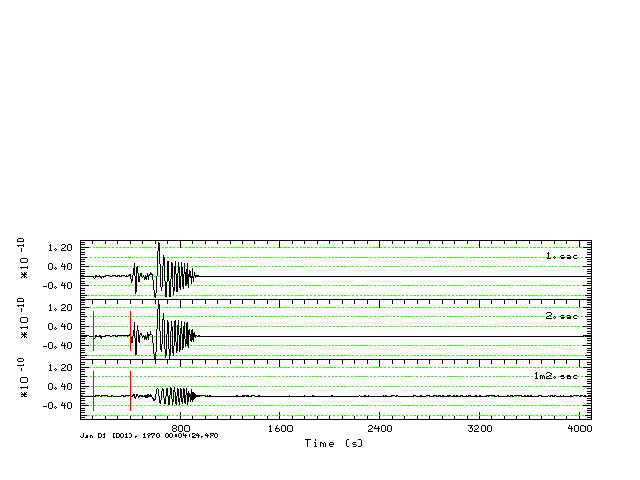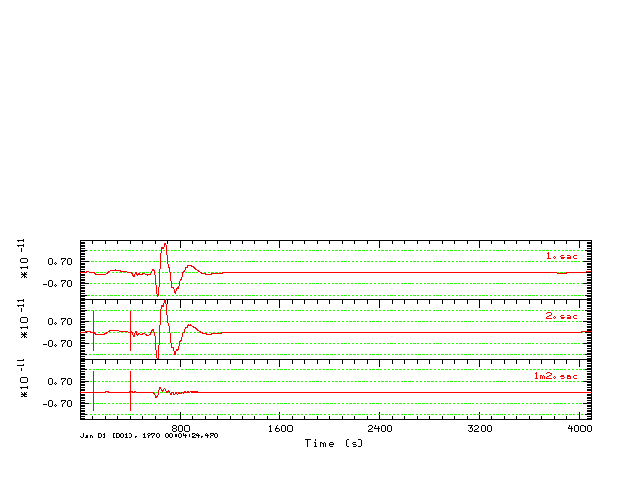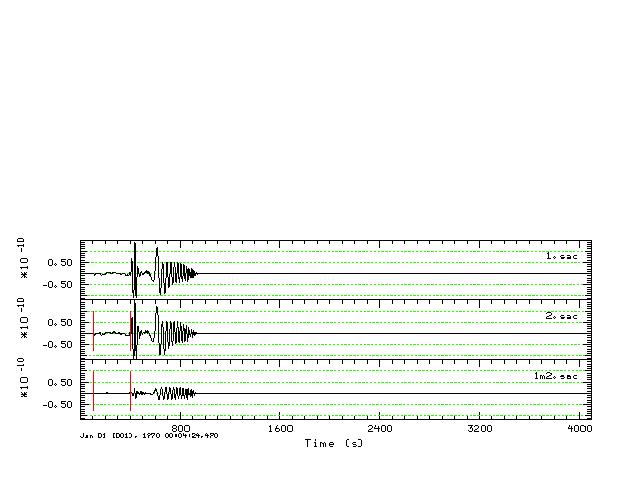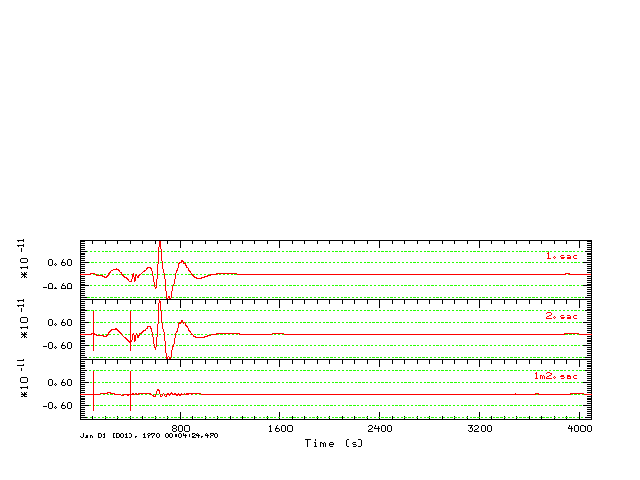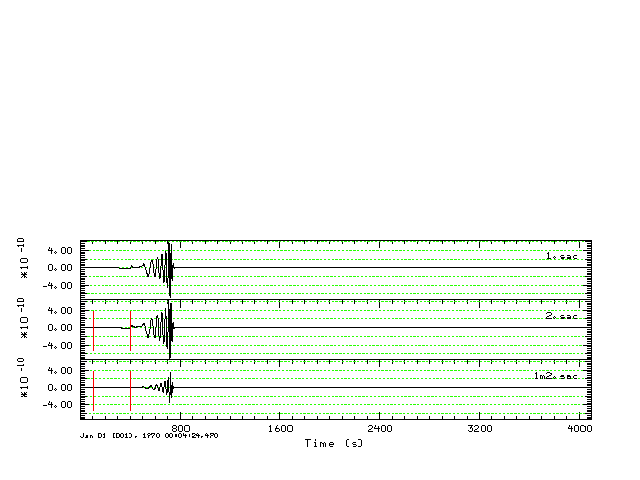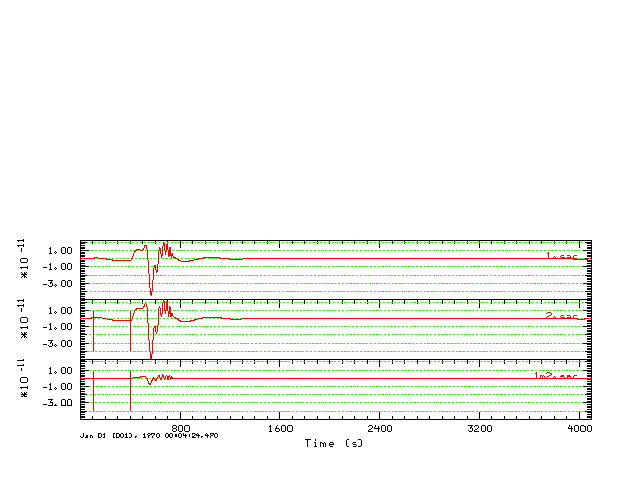010 060 SS `Model: ak135Distance Depth Phase Travel Ray Param Purist Purist (deg) (km) Name Time (s) p (s/deg) Distance Name---------------------------------------------------------------- 60.00 10.0 P 606.70 6.866 60.00 = P 60.00 10.0 pP 609.92 6.872 60.00 = pP 60.00 10.0 sP 611.13 6.870 60.00 = sP 60.00 10.0 PcP 652.77 4.000 60.00 = PcP 60.00 10.0 pPcP 656.14 3.999 60.00 = pPcP 60.00 10.0 PP 738.99 8.843 60.00 = PP 60.00 10.0 PPP 820.84 10.896 60.00 = PPP 60.00 10.0 PPP 825.91 11.848 60.00 = PPP 60.00 10.0 PPP 826.62 11.508 60.00 = PPP 60.00 10.0 PPP 837.11 9.226 60.00 = PPP 60.00 10.0 PPP 838.07 9.486 60.00 = PPP 60.00 10.0 ScP 893.85 4.436 60.00 = ScP 60.00 10.0 PcS 895.04 4.436 60.00 = PcS 60.00 10.0 pPcS 898.39 4.436 60.00 = pPcS 60.00 10.0 S 1099.21 12.858 60.00 = S 60.00 10.0 pS 1103.14 12.871 60.00 = pS 60.00 10.0 sS 1104.51 12.866 60.00 = sS 60.00 10.0 ScS 1197.34 7.442 60.00 = ScS 60.00 10.0 SS 1335.71 15.691 60.00 = SS 60.00 10.0 sSS 1340.76 15.693 60.00 = sSS 60.00 10.0 SSS 1497.04 19.990 60.00 = SSS 60.00 10.0 SSS 1501.76 24.098 60.00 = SSS 60.00 10.0 SSS 1502.67 22.630 60.00 = SSS 60.00 10.0 SSS 1504.28 23.642 60.00 = SSS 60.00 10.0 SSS 1508.13 21.287 60.00 = SSS 60.00 10.0 SSS 1522.54 16.642 60.00 = SSS 60.00 10.0 SSS 1525.60 17.313 60.00 = SSS 60.00 10.0 SSS 4565.72 8.343 300.00 = SSS `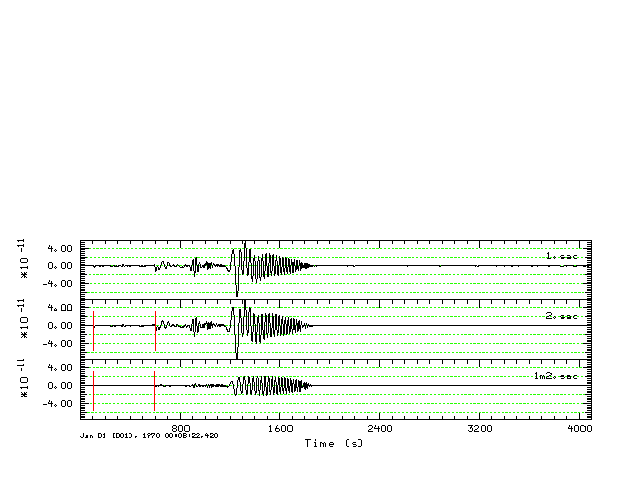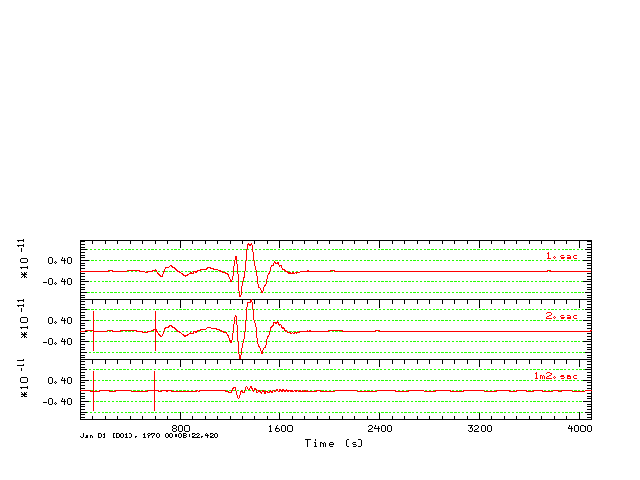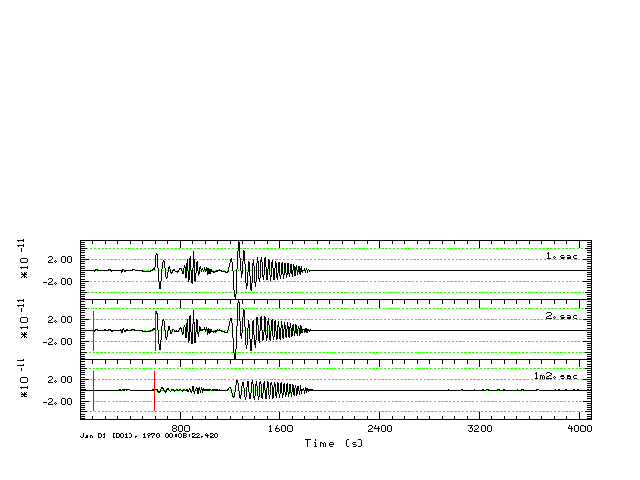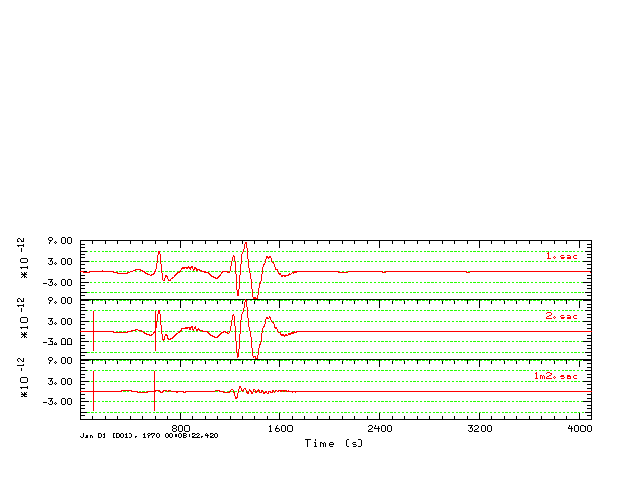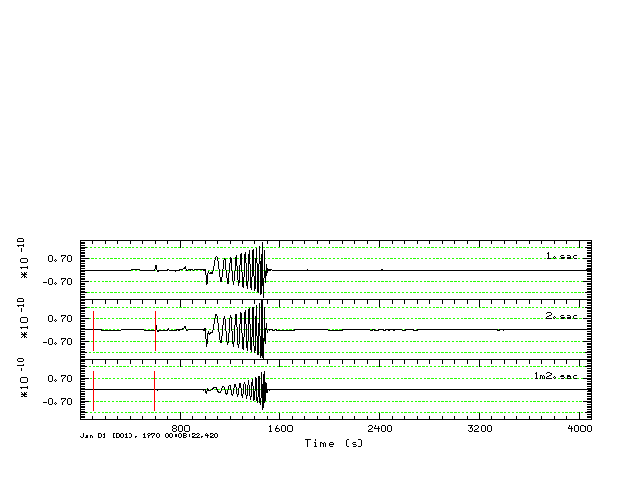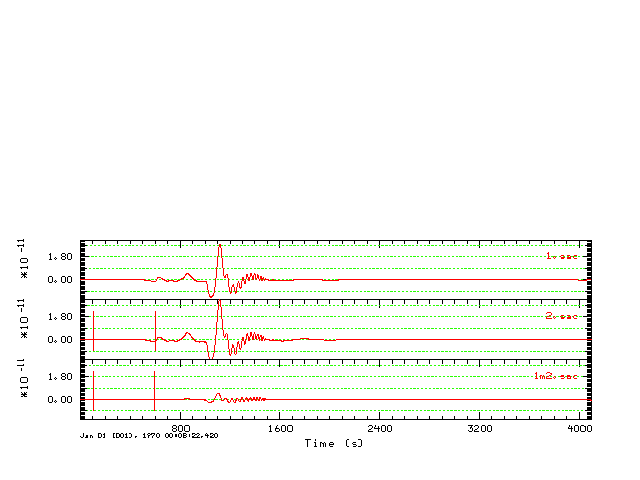010 090 SS `Model: ak135Distance Depth Phase Travel Ray Param Purist Purist (deg) (km) Name Time (s) p (s/deg) Distance Name---------------------------------------------------------------- 90.00 10.0 P 779.71 4.642 90.00 = P 90.00 10.0 PcP 780.94 4.428 90.00 = PcP 90.00 10.0 pP 783.06 4.642 90.00 = pP 90.00 10.0 sP 784.25 4.642 90.00 = sP 90.00 10.0 pPcP 784.30 4.427 90.00 = pPcP 90.00 10.0 PP 992.62 7.959 90.00 = PP 90.00 10.0 PPP 1109.25 8.843 90.00 = PPP 90.00 10.0 SKS 1410.63 5.818 90.00 = SKS 90.00 10.0 S 1432.65 9.267 90.00 = S 90.00 10.0 ScS 1436.78 8.298 90.00 = ScS 90.00 10.0 pS 1436.93 9.277 90.00 = pS 90.00 10.0 sS 1438.19 9.274 90.00 = sS 90.00 10.0 SS 1790.59 14.483 90.00 = SS 90.00 10.0 sSS 1795.75 14.488 90.00 = sSS 90.00 10.0 SSS 2004.83 15.692 90.00 = SSS 90.00 10.0 PPP 2342.49 4.642 270.00 = PPP 90.00 10.0 SSS 4303.49 9.269 270.00 = SSS `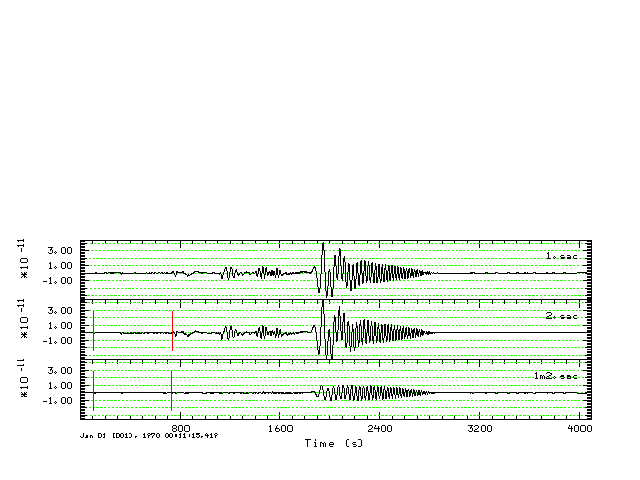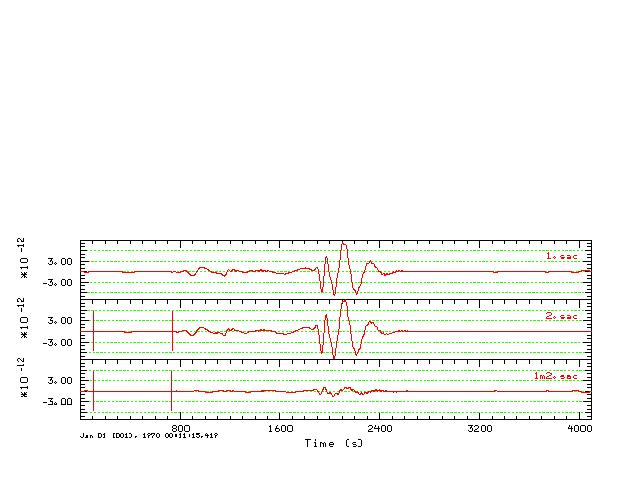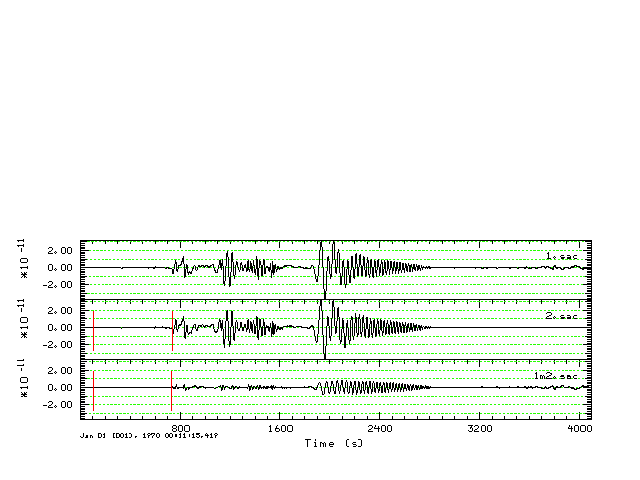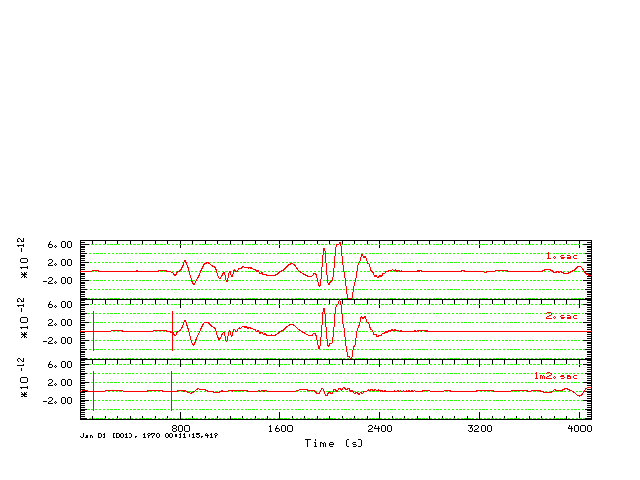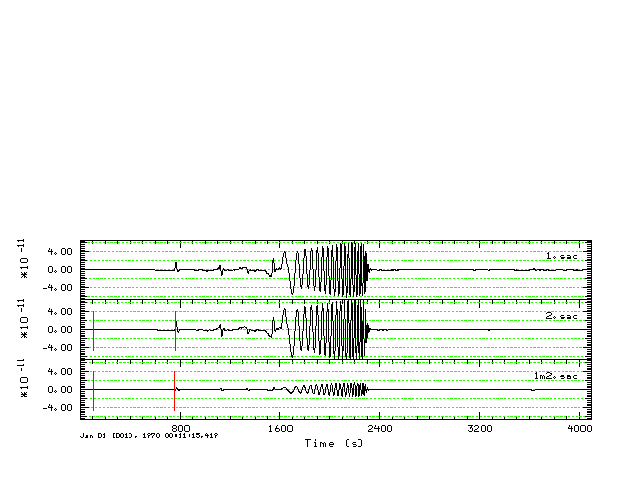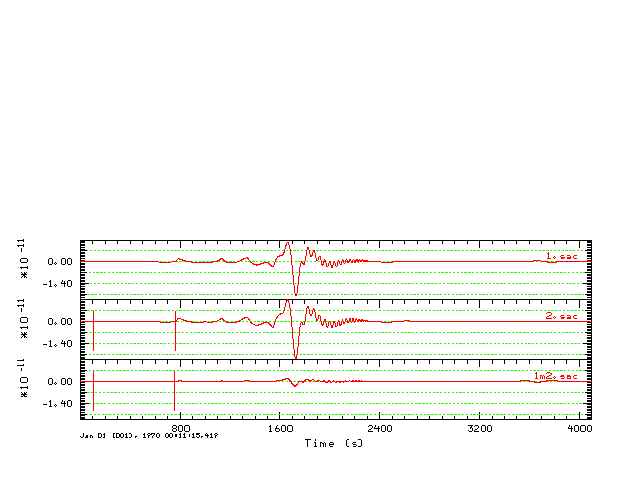100 030 SS `Model: ak135Distance Depth Phase Travel Ray Param Purist Purist (deg) (km) Name Time (s) p (s/deg) Distance Name---------------------------------------------------------------- 30.00 100.0 P 359.07 8.831 30.00 = P 30.00 100.0 pP 381.45 8.856 30.00 = pP 30.00 100.0 sP 393.03 8.850 30.00 = sP 30.00 100.0 PP 420.20 13.003 30.00 = PP 30.00 100.0 PP 421.81 13.573 30.00 = PP 30.00 100.0 PP 421.84 13.550 30.00 = PP 30.00 100.0 PP 428.54 11.105 30.00 = PP 30.00 100.0 PP 428.82 11.284 30.00 = PP 30.00 100.0 PcP 538.94 2.595 30.00 = PcP 30.00 100.0 pPcP 566.20 2.573 30.00 = pPcP 30.00 100.0 S 649.68 15.667 30.00 = S 30.00 100.0 sS 688.55 15.718 30.00 = sS 30.00 100.0 ScP 751.94 3.251 30.00 = ScP 30.00 100.0 SS 752.39 24.238 30.00 = SS 30.00 100.0 PcS 762.41 3.258 30.00 = PcS 30.00 100.0 SS 781.22 20.463 30.00 = SS 30.00 100.0 SS 781.63 20.774 30.00 = SS 30.00 100.0 pPcS 789.44 3.226 30.00 = pPcS 30.00 100.0 sSS 811.53 20.479 30.00 = sSS 30.00 100.0 sSS 811.53 20.501 30.00 = sSS 30.00 100.0 ScS 987.50 4.797 30.00 = ScS `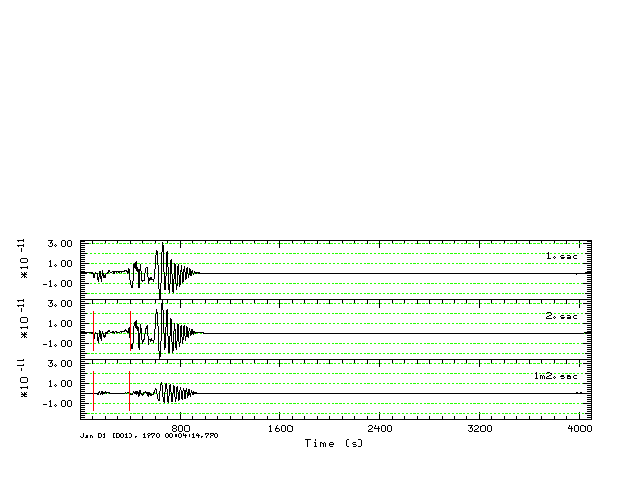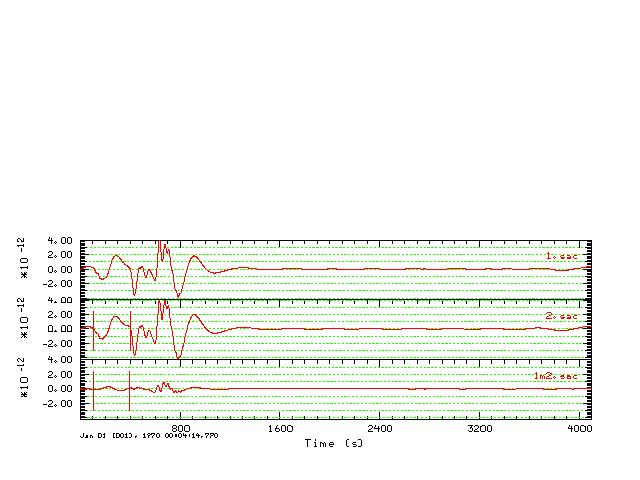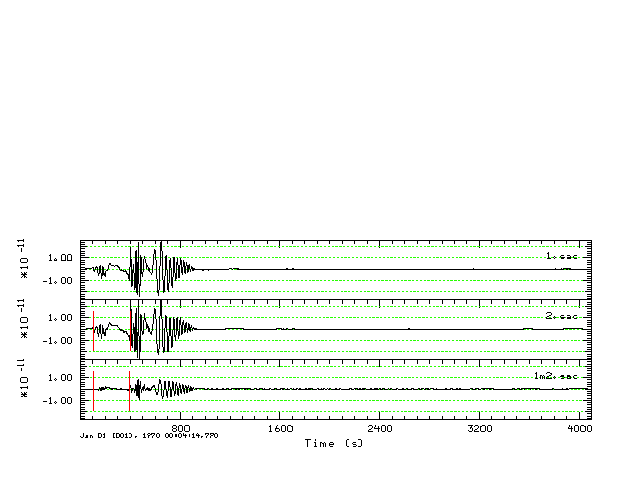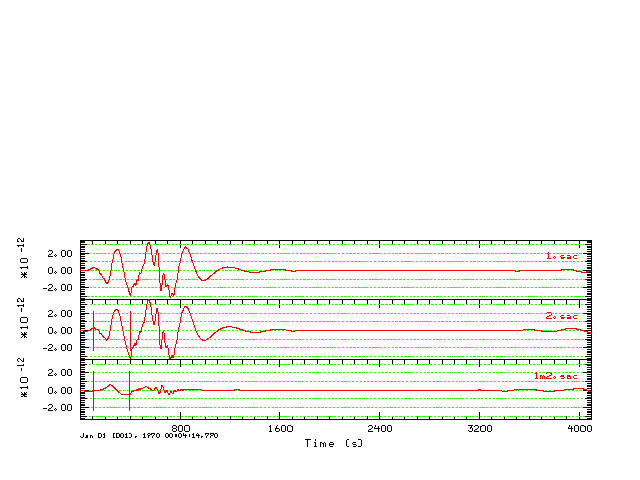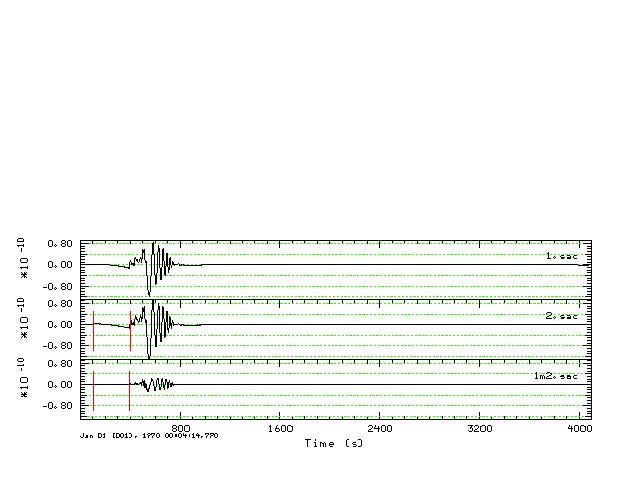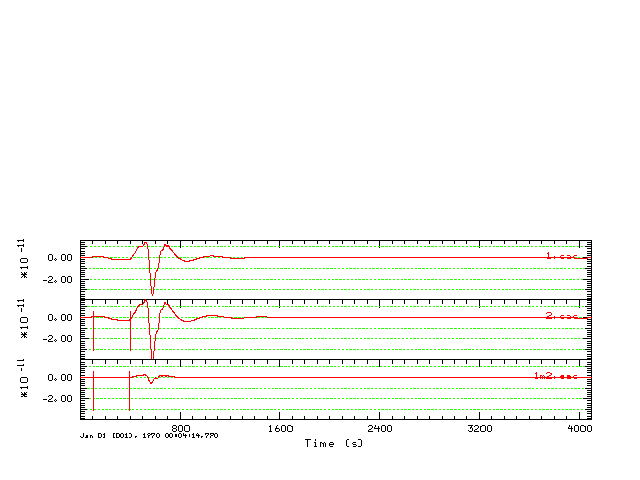100 060 SS `Model: ak135Distance Depth Phase Travel Ray Param Purist Purist (deg) (km) Name Time (s) p (s/deg) Distance Name---------------------------------------------------------------- 60.00 100.0 P 595.98 6.835 60.00 = P 60.00 100.0 pP 620.62 6.903 60.00 = pP 60.00 100.0 sP 631.65 6.887 60.00 = sP 60.00 100.0 PcP 641.11 4.007 60.00 = PcP 60.00 100.0 pPcP 667.81 3.992 60.00 = pPcP 60.00 100.0 PP 729.32 8.837 60.00 = PP 60.00 100.0 PPP 812.78 10.870 60.00 = PPP 60.00 100.0 PPP 818.80 11.755 60.00 = PPP 60.00 100.0 PPP 819.18 11.514 60.00 = PPP 60.00 100.0 PPP 827.69 9.223 60.00 = PPP 60.00 100.0 PPP 828.86 9.504 60.00 = PPP 60.00 100.0 ScP 872.90 4.437 60.00 = ScP 60.00 100.0 PcS 883.49 4.438 60.00 = PcS 60.00 100.0 pPcS 909.94 4.435 60.00 = pPcS 60.00 100.0 S 1080.74 12.810 60.00 = S 60.00 100.0 pS 1108.42 13.125 60.00 = pS 60.00 100.0 pS 1108.94 13.587 60.00 = pS 60.00 100.0 sS 1122.96 12.918 60.00 = sS 60.00 100.0 ScS 1176.96 7.455 60.00 = ScS 60.00 100.0 SS 1318.80 15.679 60.00 = SS 60.00 100.0 sSS 1357.67 15.705 60.00 = sSS 60.00 100.0 SSS 1483.54 19.917 60.00 = SSS 60.00 100.0 SSS 1492.41 22.368 60.00 = SSS 60.00 100.0 SSS 1494.96 24.033 60.00 = SSS 60.00 100.0 SSS 1496.17 21.311 60.00 = SSS 60.00 100.0 SSS 1496.40 23.776 60.00 = SSS 60.00 100.0 SSS 1506.27 16.629 60.00 = SSS 60.00 100.0 SSS 1509.83 17.348 60.00 = SSS `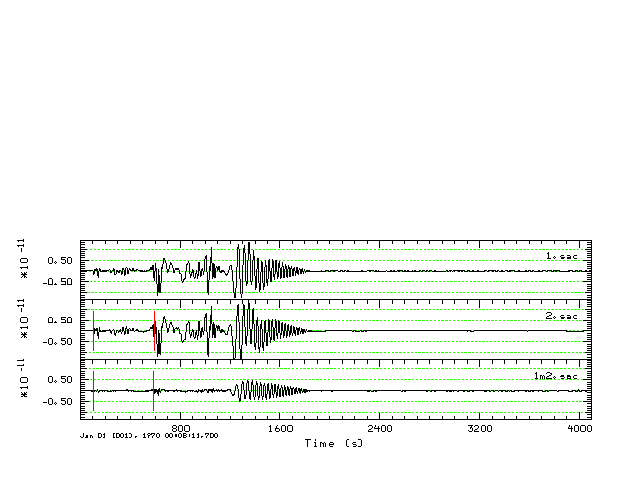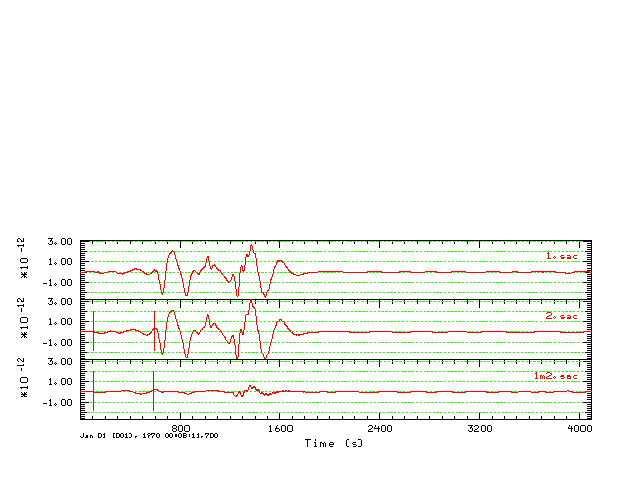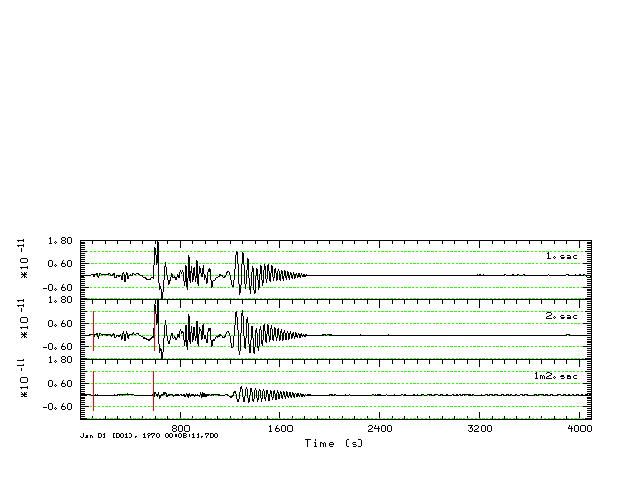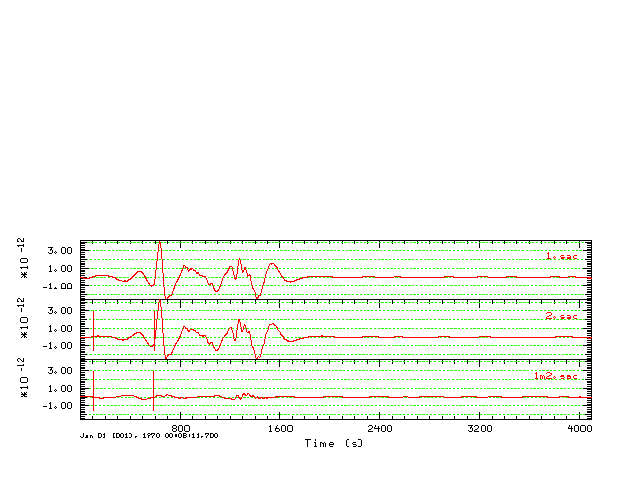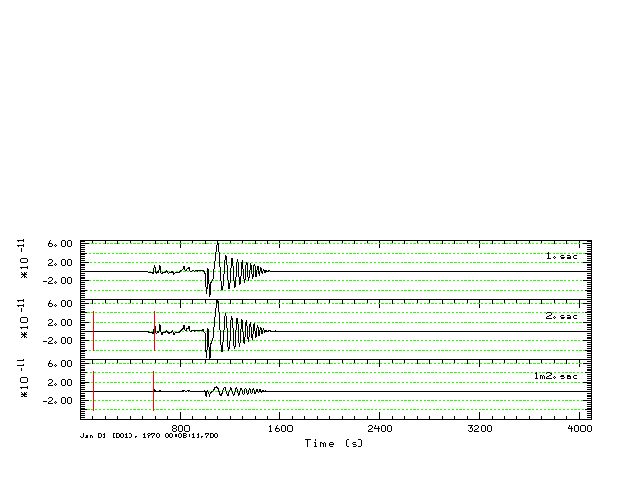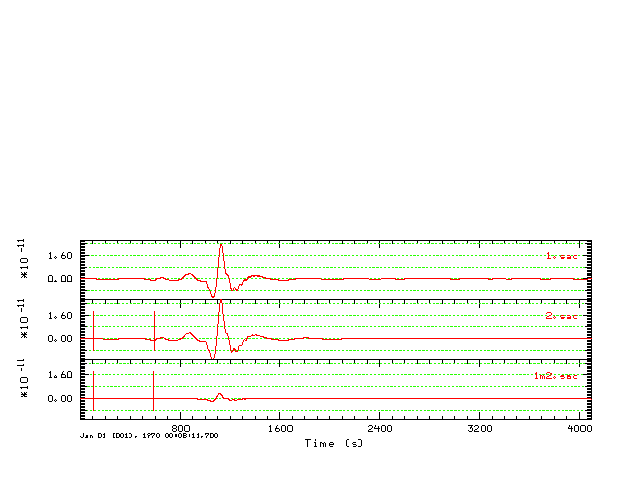100 090 SS `Model: ak135Distance Depth Phase Travel Ray Param Purist Purist (deg) (km) Name Time (s) p (s/deg) Distance Name---------------------------------------------------------------- 90.00 100.0 P 768.22 4.640 90.00 = P 90.00 100.0 PcP 769.39 4.428 90.00 = PcP 90.00 100.0 pP 794.55 4.651 90.00 = pP 90.00 100.0 pPcP 795.85 4.426 90.00 = pPcP 90.00 100.0 sP 805.17 4.643 90.00 = sP 90.00 100.0 PP 982.43 7.939 90.00 = PP 90.00 100.0 PPP 1099.58 8.839 90.00 = PPP 90.00 100.0 SKS 1389.91 5.799 90.00 = SKS 90.00 100.0 S 1412.78 9.226 90.00 = S 90.00 100.0 ScS 1416.63 8.301 90.00 = ScS 90.00 100.0 pS 1446.27 9.370 90.00 = pS 90.00 100.0 sS 1458.04 9.318 90.00 = sS 90.00 100.0 SS 1772.96 14.454 90.00 = SS 90.00 100.0 sSS 1813.37 14.517 90.00 = sSS 90.00 100.0 SSS 1987.92 15.684 90.00 = SSS 90.00 100.0 PPP 2330.99 4.641 270.00 = PPP 90.00 100.0 SSS 4283.63 9.256 270.00 = SSS `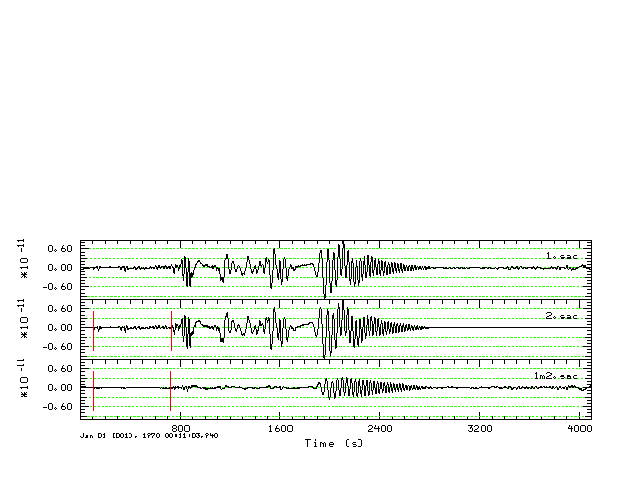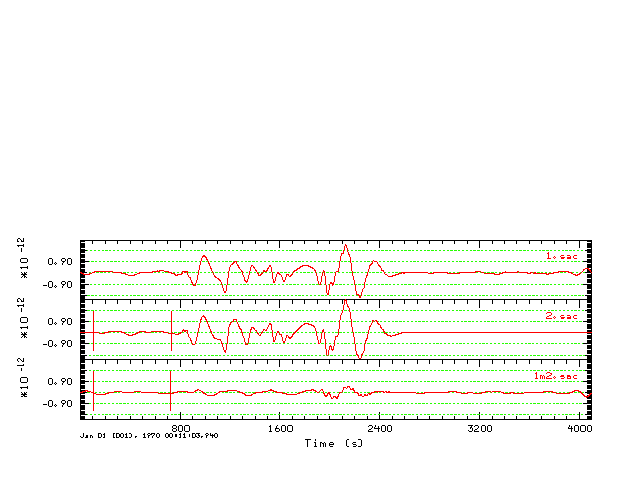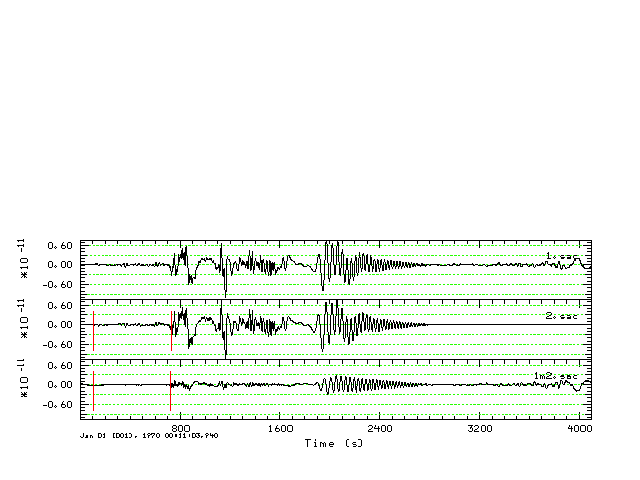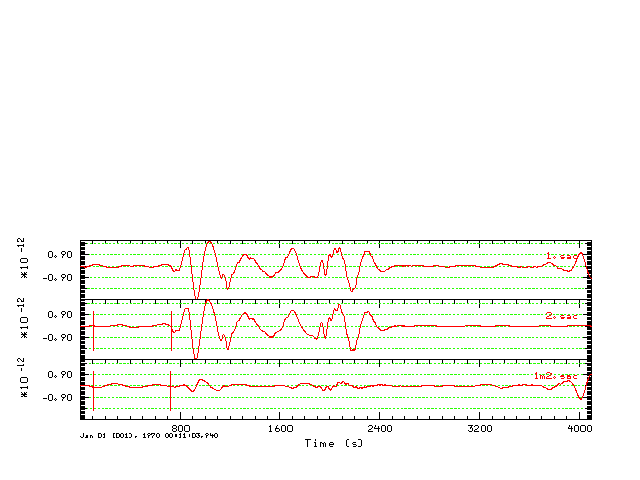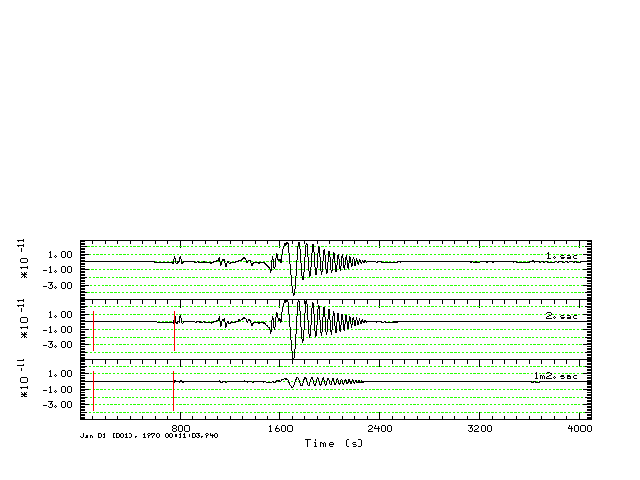700 030 SS `Model: ak135Distance Depth Phase Travel Ray Param Purist Purist (deg) (km) Name Time (s) p (s/deg) Distance Name---------------------------------------------------------------- 30.00 700.0 P 317.19 8.458 30.00 = P 30.00 700.0 PcP 474.89 2.691 30.00 = PcP 30.00 700.0 sP 501.77 9.008 30.00 = sP 30.00 700.0 sP 505.32 10.063 30.00 = sP 30.00 700.0 sP 505.56 9.764 30.00 = sP 30.00 700.0 S 570.32 15.200 30.00 = S 30.00 700.0 pPcP 630.41 2.482 30.00 = pPcP 30.00 700.0 ScP 632.98 3.323 30.00 = ScP 30.00 700.0 PcS 699.45 3.396 30.00 = PcS 30.00 700.0 pPcS 852.69 3.093 30.00 = pPcS 30.00 700.0 ScS 870.41 4.978 30.00 = ScS `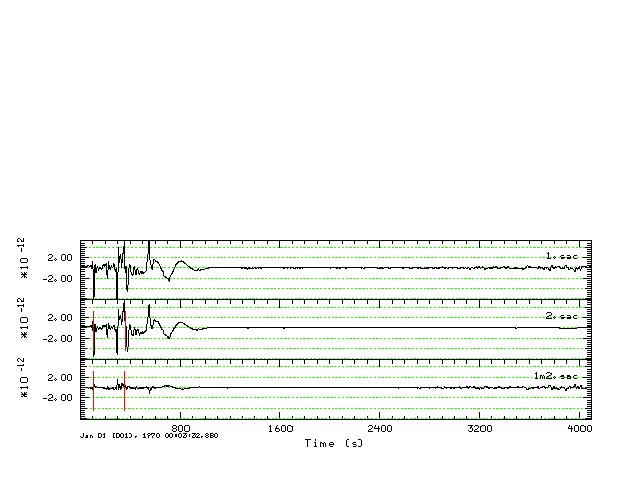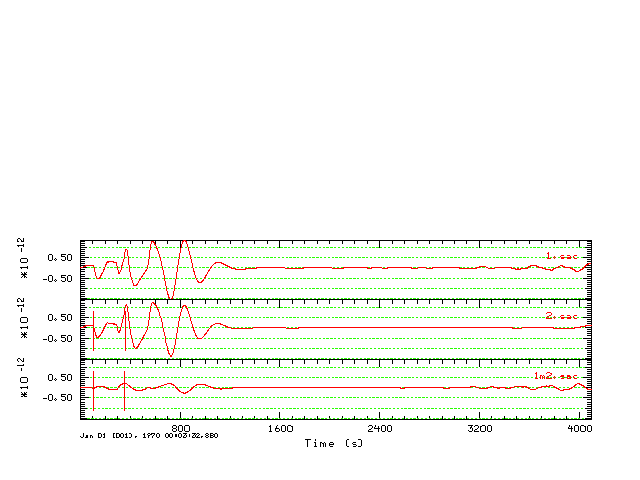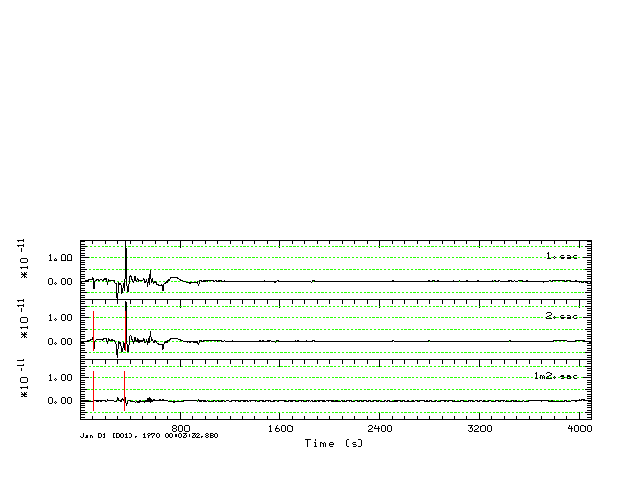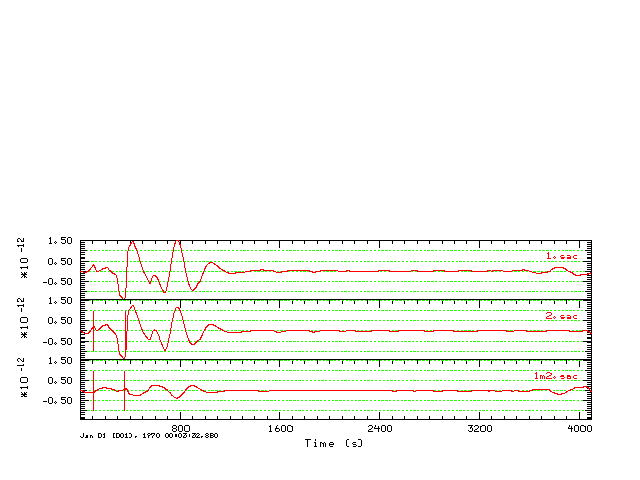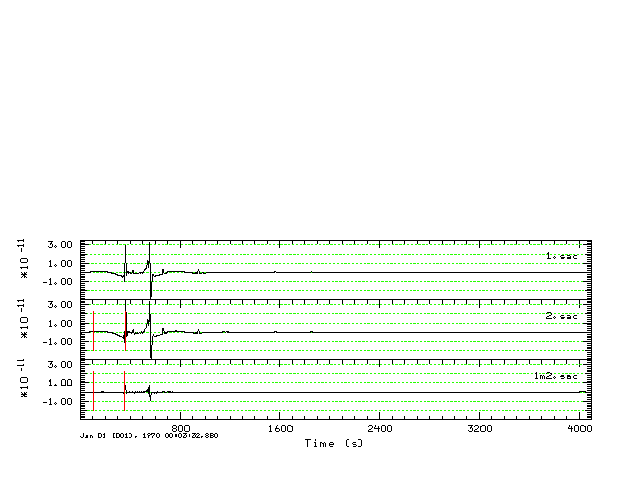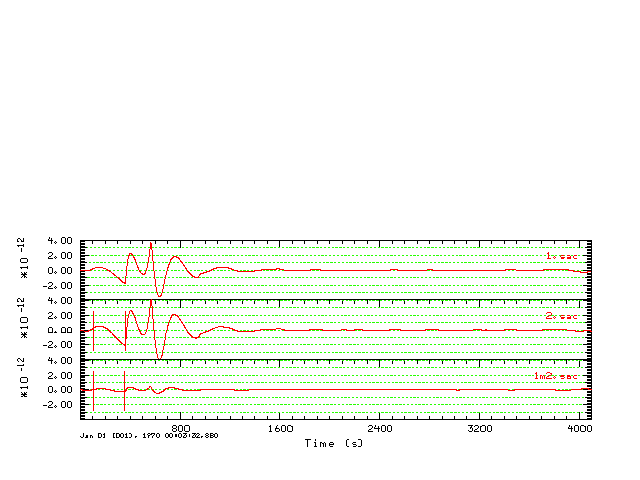700 060 SS `Model: ak135Distance Depth Phase Travel Ray Param Purist Purist (deg) (km) Name Time (s) p (s/deg) Distance Name---------------------------------------------------------------- 60.00 700.0 P 542.85 6.535 60.00 = P 60.00 700.0 PcP 579.56 4.065 60.00 = PcP 60.00 700.0 pP 671.94 7.270 60.00 = pP 60.00 700.0 PP 688.32 8.677 60.00 = PP 60.00 700.0 pPcP 729.50 3.930 60.00 = pPcP 60.00 700.0 sP 745.13 7.036 60.00 = sP 60.00 700.0 ScP 755.20 4.442 60.00 = ScP 60.00 700.0 PcS 822.90 4.444 60.00 = PcS 60.00 700.0 pPcS 970.57 4.415 60.00 = pPcS 60.00 700.0 S 985.04 12.314 60.00 = S 60.00 700.0 ScS 1064.67 7.571 60.00 = ScS 60.00 700.0 sS 1215.61 13.494 60.00 = sS 60.00 700.0 SS 1240.46 15.477 60.00 = SS 60.00 700.0 sSS 1434.78 15.880 60.00 = sSS 60.00 700.0 SSS 1435.40 16.256 60.00 = SSS `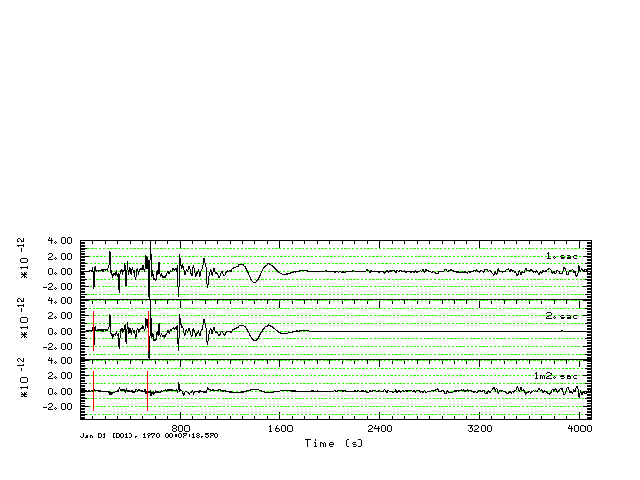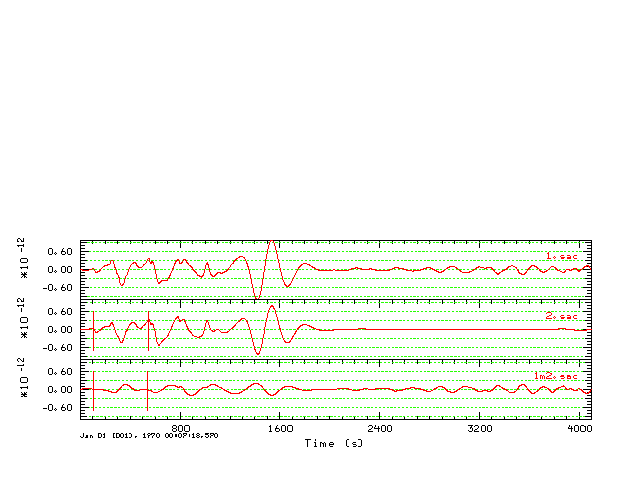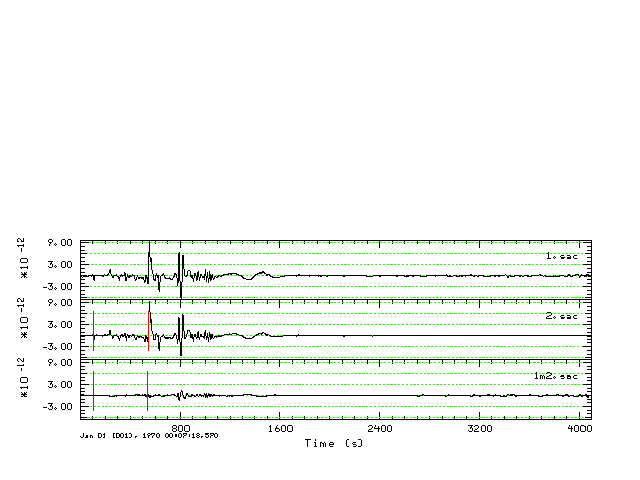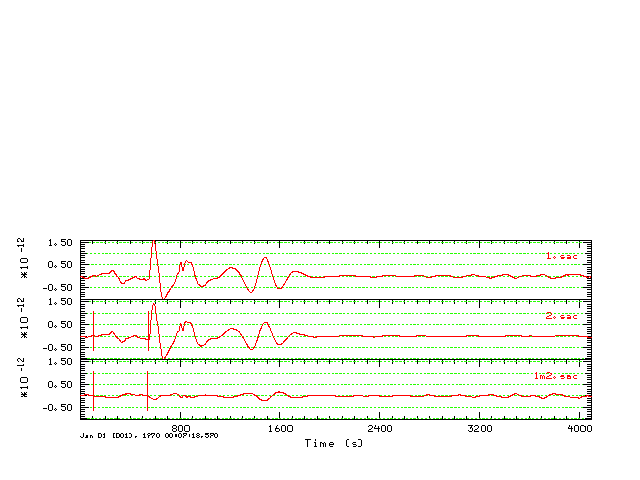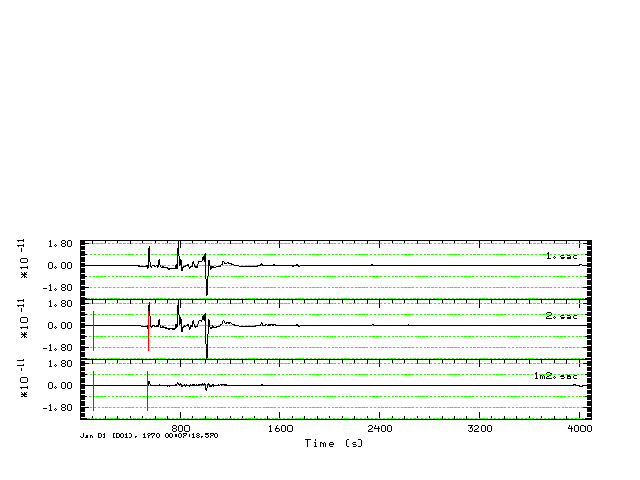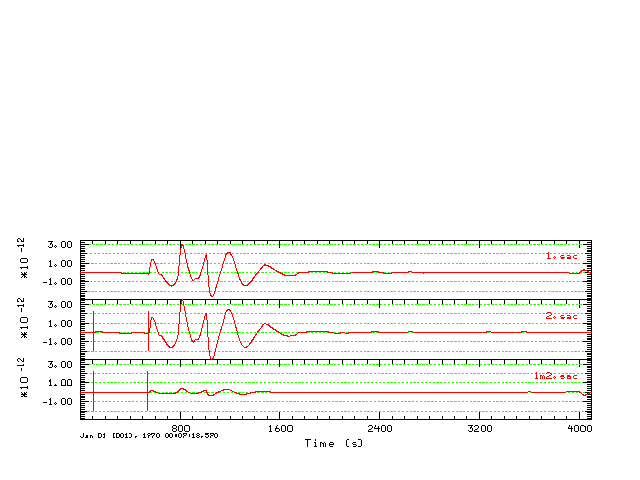700 090 SS `Model: ak135Distance Depth Phase Travel Ray Param Purist Purist (deg) (km) Name Time (s) p (s/deg) Distance Name---------------------------------------------------------------- 90.00 700.0 P 708.11 4.615 90.00 = P 90.00 700.0 PcP 708.78 4.437 90.00 = PcP 90.00 700.0 pP 854.31 4.870 90.00 = pP 90.00 700.0 pPcP 856.50 4.415 90.00 = pPcP 90.00 700.0 sP 922.52 4.749 90.00 = sP 90.00 700.0 PP 935.08 7.735 90.00 = PP 90.00 700.0 PPP 1058.84 8.749 90.00 = PPP 90.00 700.0 SKS 1274.09 5.638 90.00 = SKS 90.00 700.0 S 1304.35 8.849 90.00 = S 90.00 700.0 ScS 1306.25 8.319 90.00 = ScS 90.00 700.0 sS 1565.06 9.731 90.00 = sS 90.00 700.0 SS 1686.22 14.159 90.00 = SS 90.00 700.0 sSS 1897.89 14.832 90.00 = sSS 90.00 700.0 SSS 1909.89 15.570 90.00 = SSS 90.00 700.0 PPP 2270.91 4.637 270.00 = PPP 90.00 700.0 SSS 4175.64 9.121 270.00 = SSS `